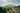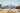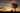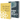# How to React Range

by Robin WieruchThis tutorial is part 2 of 2 in the series.

In this React component tutorial by example, we will create a React Range Component with and a . You can see the final output of this implementation in this CodeSandbox or in this GitHub repository. If you want to implement it step by step, just follow the tutorial.

## React Range: Component

We are starting off with the previous tutorial where we have implemented a React Slider Component. Let's rename all its internals from Slider/slider to Range/range to keep our naming of things consistent. This tutorial will extend the component to a Range Slider which has a couple of more features. Let's get started.

First, we want to colorize the range -- or also called track -- which is used for our interactive thumb to move from minimum to maximum of the range's capacity. But we will only colorize the part left of the thumb. This way, we get a visual feedback about which range has been selected and which not.

`... const StyledRangeProgress = styled.div`  border-radius: 3px;  position: absolute;  height: 100%;  opacity: 0.5;  background: #823eb7;`; ... const getWidth = percentage => `\${percentage}%`; const Range = ({  initial,  max,  formatFn = number => number.toFixed(0),  onChange,}) => {  const initialPercentage = getPercentage(initial, max);   const rangeRef = React.useRef();  const rangeProgressRef = React.useRef();  const thumbRef = React.useRef();  const currentRef = React.useRef();   ...   const handleMouseMove = event => {    ...     const newPercentage = getPercentage(newX, end);    const newValue = getValue(newPercentage, max);     thumbRef.current.style.left = getLeft(newPercentage);    rangeProgressRef.current.style.width = getWidth(newPercentage);    currentRef.current.textContent = formatFn(newValue);     onChange(newValue);  };   ...   return (    <>      <RangeHeader>        <strong ref={currentRef}>{formatFn(initial)}</strong>        &nbsp;/&nbsp;        {max}      </RangeHeader>      <StyledRange ref={rangeRef}>        <StyledRangeProgress          style={{ width: getWidth(initialPercentage) }}          ref={rangeProgressRef}        />        <StyledThumb          style={{ left: getLeft(initialPercentage) }}          ref={thumbRef}          onMouseDown={handleMouseDown}        />      </StyledRange>    </>  );};`

Essentially we are doing four things here to update the range without React's state management:

• defining a styled Range Progress for our colorized part of the track
• creating a ref with React's useRef and using it for DOM manipulation on our rendered Range Progress
• rendering this new Range Progress with an initial width coming from our calculated percentage (declarative)
• using the ref when our mouse event fires to set the new width of the Range Progress (imperative)

Next, we are going to introduce a minimum (`min`) value next to our already familiar maximum (`max`) value. This way, we are not always counting from 0 to maximum, but can choose to have two dynamic values (min and max) for our range. If no minimum value is set for our Range component, we will default to zero.

`... const RangeHeader = styled.div`  display: flex;  justify-content: space-between;`; ... const Range = ({  initial,  min = 0,  max,  formatFn = number => number.toFixed(0),  onChange,}) => {  ...   return (    <>      <RangeHeader>        <div>{formatFn(min)}</div>        <div>          <strong ref={currentRef}>{formatFn(initial)}</strong>          &nbsp;/&nbsp;          {formatFn(max)}        </div>      </RangeHeader>      ...    </>  );}; const App = () => (  <div>    <Range      initial={10}      min={5}      max={25}      formatFn={number => number.toFixed(2)}      onChange={value => console.log(value)}    />  </div>);`

We are showing the minimum value, but we are not using it yet for our calculations of the new `value` and `percentage` in our mouse move handler and our initial calculation for the percentage. Before we just assumed in our calculations that our minimum to be zero. Let's change this by taking the `min` into account for our value and percentage calculations:

`... const getPercentage = (current, min, max) =>  ((current - min) / (max - min)) * 100; const getValue = (percentage, min, max) =>  ((max - min) / 100) * percentage + min; ... const Range = ({  initial,  min = 0,  max,  formatFn = number => number.toFixed(0),  onChange,}) => {  const initialPercentage = getPercentage(initial, min, max);   ...   const handleMouseMove = event => {    ...     const newPercentage = getPercentage(newX, start, end);    const newValue = getValue(newPercentage, min, max);     thumbRef.current.style.left = getLeft(newPercentage);    rangeProgressRef.current.style.width = getWidth(newPercentage);    currentRef.current.textContent = formatFn(newValue);     onChange(newValue);  };   ...};`

When interacting with the Range component's thumb, you will notice that the track's progress, the thumb's position, and the current value are correct -- even though the `min` value isn't zero. The current shown value shouldn't go below the defined `min` value.

Next, we will do a refactoring for our React Range component. So far, everything is initialized once when our component renders for the first time. We are doing it the declarative way with our JSX -- that's how React taught us at least how to do it:

`const Range = ({ ... }) => {  ...   return (    <>      <RangeHeader>        <div>{formatFn(min)}</div>        <div>          <strong ref={currentRef}>{formatFn(initial)}</strong>          &nbsp;/&nbsp;          {formatFn(max)}        </div>      </RangeHeader>      <StyledRange ref={rangeRef}>        <StyledRangeProgress          style={{ width: getWidth(initialPercentage) }}          ref={rangeProgressRef}        />        <StyledThumb          style={{ left: getLeft(initialPercentage) }}          ref={thumbRef}          onMouseDown={handleMouseDown}        />      </StyledRange>    </>  );};`

However, since we are already using the imperative way to update all of these values once someone moves the range in our component, we could use the imperative way of doing things for the initial rendering as well. Let's remove the JSX for the initial rendering and use a React Hook instead to trigger the update function imperatively.

First, let's move everything that needs to be updated to its own function:

`const Range = ({ ... }) => {  ...   const handleUpdate = (value, percentage) => {    thumbRef.current.style.left = getLeft(percentage);    rangeProgressRef.current.style.width = getWidth(percentage);    currentRef.current.textContent = formatFn(value);  };   const handleMouseMove = event => {    ...     const newPercentage = getPercentage(newX, start, end);    const newValue = getValue(newPercentage, min, max);     handleUpdate(newValue, newPercentage);     onChange(newValue);  };   ...};`

Second, let's remove the declarative JSX and replace it with a React useLayoutEffect Hook that runs with the first rendering of the component (and on every dependency change) to update all displayed values with our previously extracted updater function:

`const Range = ({ ... }) => {  const initialPercentage = getPercentage(initial, min, max);   const rangeRef = React.useRef();  const rangeProgressRef = React.useRef();  const thumbRef = React.useRef();  const currentRef = React.useRef();   const diff = React.useRef();   const handleUpdate = (value, percentage) => {    thumbRef.current.style.left = getLeft(percentage);    rangeProgressRef.current.style.width = getWidth(percentage);    currentRef.current.textContent = formatFn(value);  };   const handleMouseMove = event => { ... };   const handleMouseUp = () => { ... };   const handleMouseDown = event => { ... };   React.useLayoutEffect(() => {    handleUpdate(initial, initialPercentage);  }, [initial, initialPercentage, handleUpdate]);   return (    <>      <RangeHeader>        <div>{formatFn(min)}</div>        <div>          <strong ref={currentRef} />          &nbsp;/&nbsp;          {formatFn(max)}        </div>      </RangeHeader>      <StyledRange ref={rangeRef}>        <StyledRangeProgress ref={rangeProgressRef} />        <StyledThumb ref={thumbRef} onMouseDown={handleMouseDown} />      </StyledRange>    </>  );};`

Now we run this React hook on the first render and every time one of its dependencies changes -- hence the second array as argument -- to handle the update imperatively instead of relying on JSX.

Last, we need to wrap our update function into React's useCallback hook, otherwise the update function would change on every render and run our useLayoutEffect hook again and again. The `handleUpdate` function should only be re-defined when one of its dependencies (here `formatFn`) changes.

The 'handleUpdate' function makes the dependencies of useLayoutEffect Hook change on every render. To fix this, wrap the 'handleUpdate' definition into its own useCallback() Hook.

`const Range = ({ ... }) => {  ...   const handleUpdate = React.useCallback(    (value, percentage) => {      thumbRef.current.style.left = getLeft(percentage);      rangeProgressRef.current.style.width = getWidth(percentage);      currentRef.current.textContent = formatFn(value);    },    [formatFn]  );   ...   React.useLayoutEffect(() => {    handleUpdate(initial, initialPercentage);  }, [initial, initialPercentage, handleUpdate]);   ...};`

Everything should work again. However, keep in mind that it's recommended to avoid the imperative way of doing things in React. So see this as an exercise to move things from declarative (JSX) to imperative (useRef) programming -- since we needed the imperative programming anyway for updating everything on our mouse move event without using React's state management. In the future, try to stick to React's declarative way of doing things for state management and displaying values.

### Exercises:

• Give your Range Component a disabled state where it's no longer possible to interact with it.
• Add a second thumb to the Range Component for being able to select a part within the track which doesn't start with our defined `min` value.

The React Range Component was inspired by this pure JavaScript implementation. Let me know in the comments how you improved your component and how you liked the tutorial.## How to React Slider

In this React component tutorial by example, we will create a React Slider Component with React Hooks and a Function Component . You can see the final output of this implementation in this…## How to use React Testing Library Tutorial

React Testing Library (RTL) by Kent C. Dodds got released as alternative to Airbnb's Enzyme . While Enzyme gives React developers utilities to test internals of React components, React Testing…